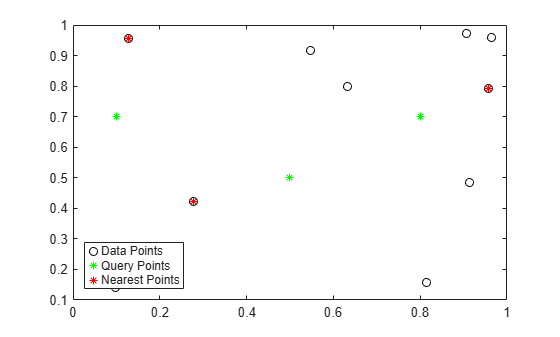# dsearchn

Nearest point search

## Syntax

``k = dsearchn(P,PQ)``
``k = dsearchn(P,T,PQ)``
``k = dsearchn(P,T,PQ,outind)``
``[k,dist] = dsearchn(___)``

## Description

example

````k = dsearchn(P,PQ)` returns the indices of the closest points in `P` to the query points in `PQ` measured in Euclidean distance.```
````k = dsearchn(P,T,PQ)` returns the indices of the closest points in `P` by using the Delaunay triangulation `T`, where `T = delaunayn(P)`. Providing `T` can improve search performance when `PQ` contains a large number of points.```
````k = dsearchn(P,T,PQ,outind)` returns the indices of the closest points in `P`, but assigns an index value of `outind` for query points that are outside of the convex hull of `P`. For example, `desearchn(P,T,PQ,Inf)` returns an index value of `Inf` for query points outside of the convex hull.```

example

````[k,dist] = dsearchn(___)` also returns the distance from each point in `P` to the corresponding query point in `PQ`.```

## Examples

collapse all

Create a matrix `P` of 2-D data points and a matrix `PQ` of 2-D query points. Find the nearest data point to each query point, and compute the corresponding distances.

```rng default; P = rand([10 2]); PQ = [0.5 0.5; 0.1 0.7; 0.8 0.7]; [k,dist] = dsearchn(P,PQ);```

Plot the data points and query points, and highlight the data point nearest to each query point.

```plot(P(:,1),P(:,2),'ko') hold on plot(PQ(:,1),PQ(:,2),'*g') hold on plot(P(k,1),P(k,2),'*r') legend('Data Points','Query Points','Nearest Points','Location','sw')```Display the distances.

`dist`
```dist = 3×1 0.2349 0.2586 0.1825 ```

## Input Arguments

collapse all

Points, specified as an m-by-n matrix containing m points of dimension n. For example, `P = [0 0 0; 1 1 1]` represents the 3-D coordinates for the points (0,0,0) and (1,1,1).

Query points, specified as an r-by-n matrix containing r points of dimension n. For example, the 2-by-3 matrix `PQ = [-1 -1 -1; 2 2 2]` represents the 3-D coordinates for the two query points (-1,-1,-1) and (2,2,2).

The number of columns in `PQ` must match the number of columns in `P`.

Delaunay triangulation, specified as a matrix returned by the `delaunayn` function.

Outside index value, specified as a scalar index value for query points outside of the convex hull.

If `outval` is specified as `[]`, then the output `k` is equivalent to the syntax ```k = dsearchn(P,T,PQ)```.

## Output Arguments

collapse all

Indices, returned as a column vector containing the indices of the data points closest to the query points. The length of `k` is equal to the number of query points.

Distance, returned as a column vector containing the Euclidean distances between each query point and the closest input point. The length of `dist` is equal to the number of query points.

## Algorithms

`dsearchn` is based on Qhull . For information about Qhull, see http://www.qhull.org/. For copyright information, see http://www.qhull.org/COPYING.txt.

 Barber, C.B., D.P. Dobkin, and H.T. Huhdanpaa. “The Quickhull Algorithm for Convex Hulls.” ACM Transactions on Mathematical Software, Vol. 22, No. 4, Dec. 1996, p 469–483.